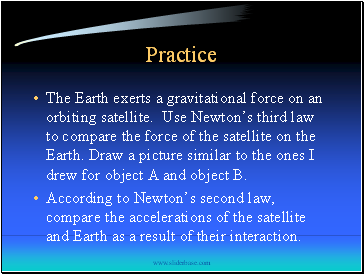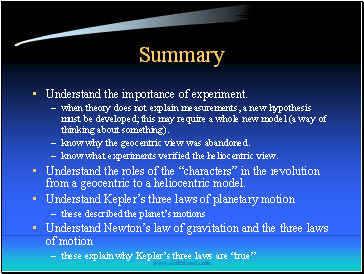# History of Modern AstronomyPage 4

#### WATCH ALL SLIDES

This is called an “inverse-square law.”

mA

mB

ForceB on A

ForceA on B

Slide 28Practice

The Earth exerts a gravitational force on an orbiting satellite. Use Newton’s third law to compare the force of the satellite on the Earth. Draw a picture similar to the ones I drew for object A and object B.

According to Newton’s second law, compare the accelerations of the satellite and Earth as a result of their interaction.

Slide 29## Deriving Kepler’s laws

Newton’s law of gravitation, and Newton’s second law (net force = mass x acceleration) can be used to derive Kepler’s three laws of planetary motion.

Slide 30Summary

Understand the importance of experiment.

when theory does not explain measurements, a new hypothesis must be developed; this may require a whole new model (a way of thinking about something).

know why the geocentric view was abandoned.

know what experiments verified the heliocentric view.

Understand the roles of the “characters” in the revolution from a geocentric to a heliocentric model.

Understand Kepler’s three laws of planetary motion

these described the planet’s motions

Understand Newton’s law of gravitation and the three laws of motion

these explain why Kepler’s three laws are “true”

Go to page:
1  2  3  4

## Last added presentations

© 2010-2021 powerpoint presentations